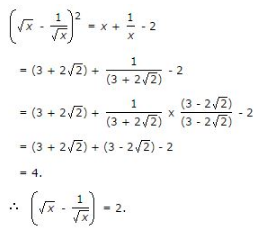# Surds & Indices - MCQ 1

## 15 Questions MCQ Test Quantitative Aptitude for Competitive Examinations | Surds & Indices - MCQ 1

Description
This mock test of Surds & Indices - MCQ 1 for Quant helps you for every Quant entrance exam. This contains 15 Multiple Choice Questions for Quant Surds & Indices - MCQ 1 (mcq) to study with solutions a complete question bank. The solved questions answers in this Surds & Indices - MCQ 1 quiz give you a good mix of easy questions and tough questions. Quant students definitely take this Surds & Indices - MCQ 1 exercise for a better result in the exam. You can find other Surds & Indices - MCQ 1 extra questions, long questions & short questions for Quant on EduRev as well by searching above.
QUESTION: 1

### (17)3.5 x (17)? = 178

Solution:

Let (17)3.5 x (17)x = 178.

Then, (17)3.5 + x = 178.

∴ 3.5 + x = 8

⇒ x = (8 – 3.5)

⇒ x = 4.5

QUESTION: 2

###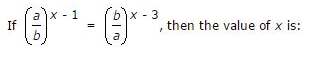Solution: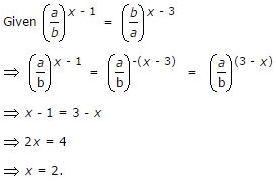QUESTION: 3

### Given that 100.48 = x, 100.70 = y and xz = y2, then the value of z is close to:

Solution: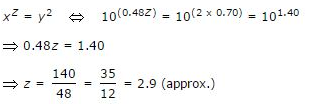QUESTION: 4

If 5a = 3125, then the value of 5(a – 3) is:

Solution:

5a = 3125    ⇔    5a = 55

⇒ a = 5.

∴ 5(a – 3) = 5(5 – 3) = 52 = 25.

QUESTION: 5

If 3(x – y) = 27 and 3(x + y) = 243, then x is equal to:

Solution:

3x – y = 27 = 33    ⇔    x – y = 3 ….(i)

3x + y = 243 = 35    ⇔    x + y = 5 ….(ii)

On solving (i) and (ii), we get x = 4.

QUESTION: 6

(256)0.16 x (256)0.09 = ?

Solution:

(256)0.16 x (256)0.09 = (256)(0.16 + 0.09)

= (256)0.25

= (256)(25/100)

= (256)(1/4)

= (44)(1/4)

= 44(1/4)

= 41

= 4

QUESTION: 7

The value of [(10)150 ÷ (10)146]

Solution: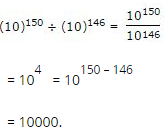QUESTION: 8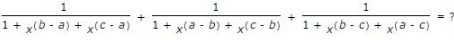Solution: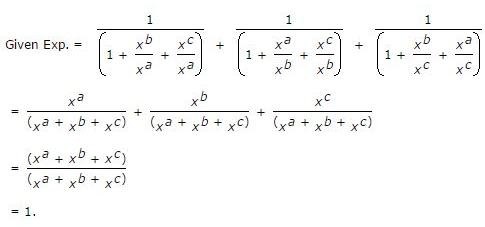QUESTION: 9

(25)7.5 x (5)2.5 ÷ (125)1.5 = 5?

Solution: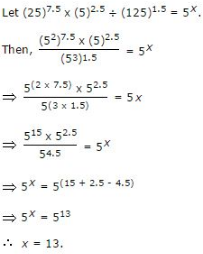QUESTION: 10

(0.04)-1.5 = ?

Solution: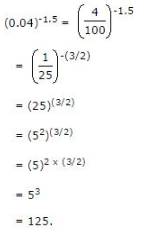QUESTION: 11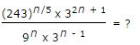Solution: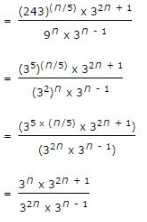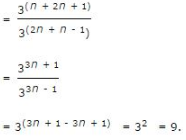QUESTION: 12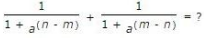Solution: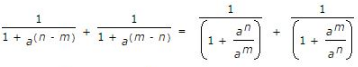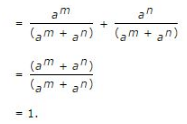QUESTION: 13

If m and n are whole numbers such that mn = 121, the value of (m – 1)n + 1 is:

Solution:

We know that 112 = 121.

Putting m = 11 and n = 2, we get:

(m – 1)n + 1 = (11 – 1)(2 + 1) = 103 = 1000.

QUESTION: 14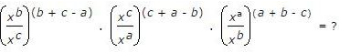Solution: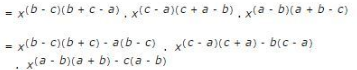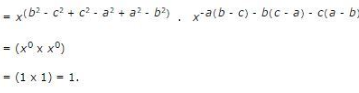QUESTION: 15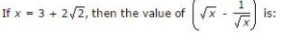Solution: